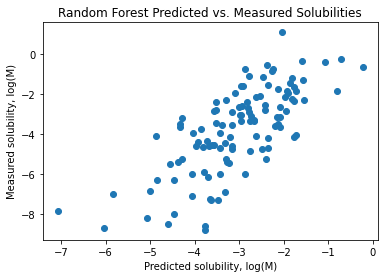# Modeling Solubility with DeepChem¶

This notebook shows how to use DeepChem to build a predictive model for aqueous solubility. It's based on one included in the DeepChem source repository. The following steps are covered:

1. Obtain a solubility dataset.
2. Inspect the dataset structures and associated data.
3. Featurize molecules.
4. Split the dataset into training, validation, and testing subsets.
5. Train a random forest model.
6. Optimize the model hyperparameters.
7. Plot prediced vs. measured solubilities for the testing subset.

Begin by importing DeepChem and ensuring the correct version.

In :
import deepchem
deepchem.__version__

Out:
'2.4.0-rc1.dev'

## Dataset¶

We'll be using an aqueous solubility dataset adapated from the paper by Delaney.

In :
%%capture
!wget https://raw.githubusercontent.com/deepchem/deepchem/master/datasets/delaney-processed.csv -O delaney-processed.csv


## Inspect¶

The next few steps will produce some simple visualizations of the data set. Only two colums will be used in this notebook:

• smiles
• measured log solubility in mols per litre

Printing column headings and row count yields a quick overview of what's contained in the data file.

In :
from deepchem.utils.save import load_from_disk

dataset_file= "delaney-processed.csv"
print("Columns: %s" % str(dataset.columns.values))
print("Rows: %s" % str(dataset.shape))

Columns: ['Compound ID' 'ESOL predicted log solubility in mols per litre'
'Minimum Degree' 'Molecular Weight' 'Number of H-Bond Donors'
'Number of Rings' 'Number of Rotatable Bonds' 'Polar Surface Area'
'measured log solubility in mols per litre' 'smiles']
Rows: 1128


To get a feel for what kinds of molecules are present, we can display the first few in a grid.

In :
from rdkit import Chem
from rdkit.Chem import Draw
from itertools import islice

molecules = [Chem.MolFromSmiles(smiles)
for smiles in islice(dataset['smiles'], 6)]

Draw.MolsToGridImage(molecules)

Out: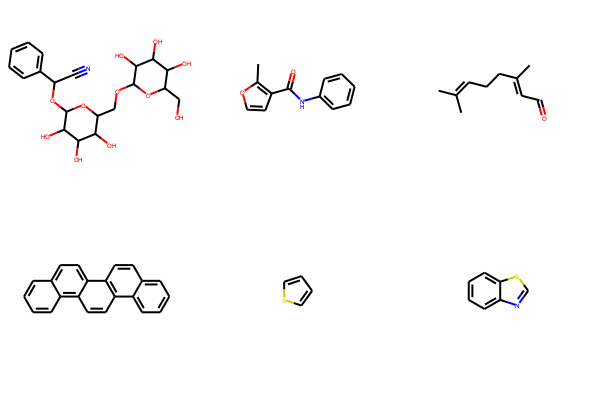That's it for structures right now.

In terms of data, we're interested in measured log(solubility). Let's plot the distribution of experimental data.

In :
%matplotlib inline
import matplotlib
import numpy as np
import matplotlib.pyplot as plt

solubilities = np.array(dataset["measured log solubility in mols per litre"])
n, bins, patches = plt.hist(solubilities, 50, facecolor='green', alpha=0.75)
plt.xlabel('Measured log-solubility in mol/liter')
plt.ylabel('Number of compounds')
plt.title(r'Histogram of solubilities')
plt.grid(True)
plt.show()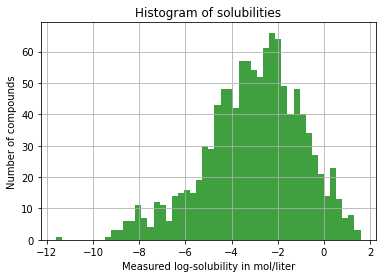## Featurization¶

Having inspected the data set, we turn to building a predictive model.

We'll need to transform the SMILES in the raw dataset into something that DeepChem can work with. Fingerprints offer a good option. Transformations of this kind are done with a DeepChem Featurizer. A Featurizer transforms input data into a form more appropriate for machine learning.

In :
import deepchem as dc

featurizer = dc.feat.CircularFingerprint(size=1024)


As the name implies, dc.feat.CircularFingerprint is a kind of featurizer that transofrms SMILES into extended connectivity (circular) fingerprints, computed by RDKit.

Having constructed the Featurizer, we rebuild dataset, replacing smiles with the corresponding fingerprint.

In :
loader = dc.data.CSVLoader(
tasks=["measured log solubility in mols per litre"], feature_field="smiles",
featurizer=featurizer)


## Split¶

It's common to split a data set into randomized training/validation/testing subsets. The DeepChem authors note that molecular datasets must further account for chemical space. Specifically, subsets of the data should attempt to generate evenly-represented populations of chemical space.

ScaffoldSplitter uses Bemis-Murcko scaffolds to perform the split. At least one other strategy is possible.

In :
splitter = dc.splits.ScaffoldSplitter()
train_dataset, valid_dataset, test_dataset = splitter.train_valid_test_split(
dataset)


The above generates three subsets, available as train_dataset, valid_dataset, and test_dataset. The first few molecules of the training set can be viewed like so:

In :
train_mols = [Chem.MolFromSmiles(compound)
for compound in train_dataset.ids]
Draw.MolsToGridImage(train_mols[:6])

Out: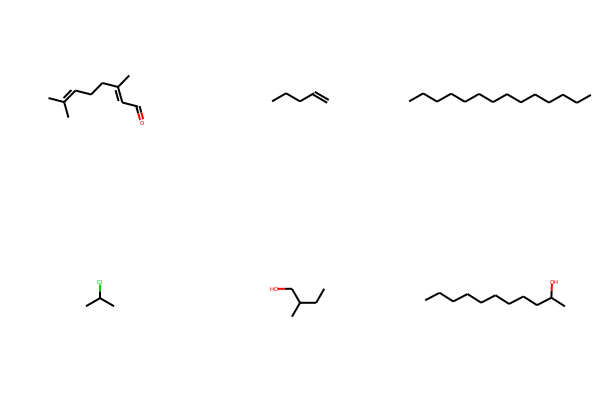And here are the first few molecules of the validation set.

In :
valid_mols = [Chem.MolFromSmiles(compound)
for compound in valid_dataset.ids]
Draw.MolsToGridImage(valid_mols[:6])

Out: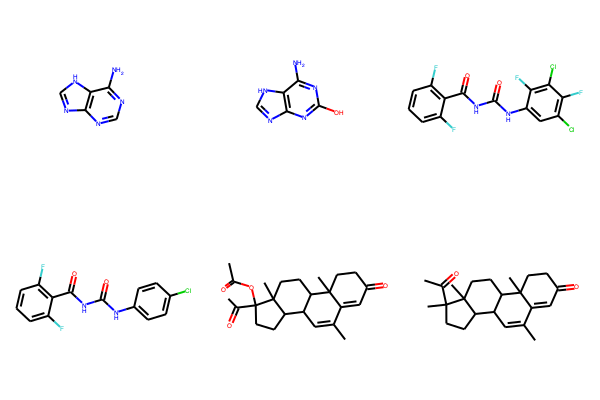One final piece of setup before training can begin training. The DeepChem authors note that performance can be sensitive to input data pre-processing. A common transofmration is to normalize it with a man of zero and unit standard deviation. NormalizationTransformer is one way to do this with DeepChem.

In :
transformers = [
dc.trans.NormalizationTransformer(transform_y=True, dataset=train_dataset)]

for dataset in [train_dataset, valid_dataset, test_dataset]:
for transformer in transformers:
dataset = transformer.transform(dataset)


## Train¶

DeepChem offers many machine learning models to choose from. In this tutorial, we'll use SciKit Learn's RandomForestRegressor. Executing this next cell could take a few seconds.

In :
from sklearn.ensemble import RandomForestRegressor

regressor = RandomForestRegressor(n_estimators=100)
model = dc.models.SklearnModel(regressor)
model.fit(train_dataset)


R2 is a common variance metric. It's available through DeepChem's Evaluator.

In :
from deepchem.utils.evaluate import Evaluator

metric = dc.metrics.Metric(dc.metrics.r2_score)
evaluator = Evaluator(model, valid_dataset, transformers)
r2score = evaluator.compute_model_performance([metric])

print(r2score)

{'r2_score': 0.1698975513661345}


## Optimize¶

Not so good. Recall that R2 of one indicates perfect correlation. A better model can be constructed by optimizing hyperparameters. We'll use two:

• n_estimators: the number of trees in the forest
• max_featurres: the number of features to consider when splitting

Here's a way to set up hyperparameter tuning:

In :
def rf_model_builder(n_estimators, max_features, model_dir):
sklearn_model = RandomForestRegressor(
n_estimators=n_estimators, max_features=max_features)
return dc.models.SklearnModel(sklearn_model, model_dir)
params_dict = {
"n_estimators": [10, 100],
"max_features": ["auto", "sqrt", "log2", None],
}

optimizer = dc.hyper.GridHyperparamOpt(rf_model_builder)
best_rf, best_rf_hyperparams, all_rf_results = optimizer.hyperparam_search(
params_dict, train_dataset, valid_dataset, transformers,
metric=metric)


This routine uses DeepChem's GridHyperparamOpt. Two other optimizations are available.

We're now ready to try again.

In :
rf_test_evaluator = Evaluator(best_rf, test_dataset, transformers)
rf_test_r2score = rf_test_evaluator.compute_model_performance([metric])
print("RF Test set R^2 %f" % (rf_test_r2score["r2_score"]))

RF Test set R^2 0.362042


Not great, but better.

## Plot¶

It's often helpful to view a scatterplot of predicted vs. measured values. Here's one way to do that:

In :
predicted_test = best_rf.predict(test_dataset)
measured_test = test_dataset.y

plt.scatter(predicted_test, measured_test)
plt.xlabel('Predicted solubility, log(M)')
plt.ylabel('Measured solubility, log(M)')
plt.title(r'Random Forest Predicted vs. Measured Solubilities')
plt.show()